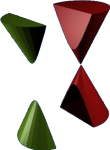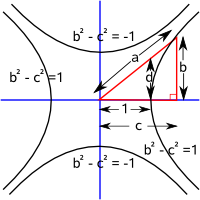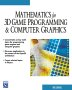# Maths - Hyperbola and Parabola

### Quadratic Equations in Two Variables

We can represent a general quadratic equation in two variables as:

A x² + B xy + C y²+ D x + E y + F = 0

In the same way that the quadratic equation in one variable:

a x² + b x + c = 0

has solutions

 x= -b ± √(b² - 4ac ) 2a

of different types depending whether:

 b² - 4ac > 0 two real solutions b² - 4ac = 0 one solution b² - 4ac < 0 complex number solutions

So our quadratic equation in two variables has different types of solution.

circle x² + y² = r²
ellipse
 x² + y² =±1 a² b²
parabola y² = 4 a x
hyperbola
 x² - y² =±1 a² b²

These types can all be visualised as conic sections.

### Equations of Hyperbola

east-west north-south
 x² - y² =1 a² b²
 x² - y² = -1 a² b²Parametric equations

x = a cosh t
y = b tanh t

x = a/cos t
y = b/tan tHyperbola Focal Points

## Equations of Parabola

y² = 4 a x

This can be represented by the intersection of the cone and a plane which is parallel to the face of the cone.## Equations of Circle and Ellipse

An ellipse is a circle that may be expanded differently in the x and y directions. Or, to reverse the argument, a circle is an ellipse whose extent is equal in both dimensions.

Circle Ellipse
x² + y² = r²
 x² + y² =±1 a² b²When we intersect the cone with a plane parallel to its base we get a circle, when we intersect at an angle (But less than the angle of the cone face) then we get an ellipse.

### Parametric equations

For comparison with above the parametric equations are:

x = a cosθ
y = b sinθ

## Conic Sections

The equation for a cone in 3 dimensions is:

(x² + y²)cos²θ - z² sin²θ

Or in terms of parametric equations:

x = u cos(θ) cos(t)
y = u cos(θ) sin(t)
z = u sin(θ)

where:

• aperture =2θ

 metadata block see also: Correspondence about this page Book Shop - Further reading. Where I can, I have put links to Amazon for books that are relevant to the subject, click on the appropriate country flag to get more details of the book or to buy it from them.Mathematics for 3D game Programming - Includes introduction to Vectors, Matrices, Transforms and Trigonometry. (But no euler angles or quaternions). Also includes ray tracing and some linear & rotational physics also collision detection (but not collision response). Other Math Books

This site may have errors. Don't use for critical systems.